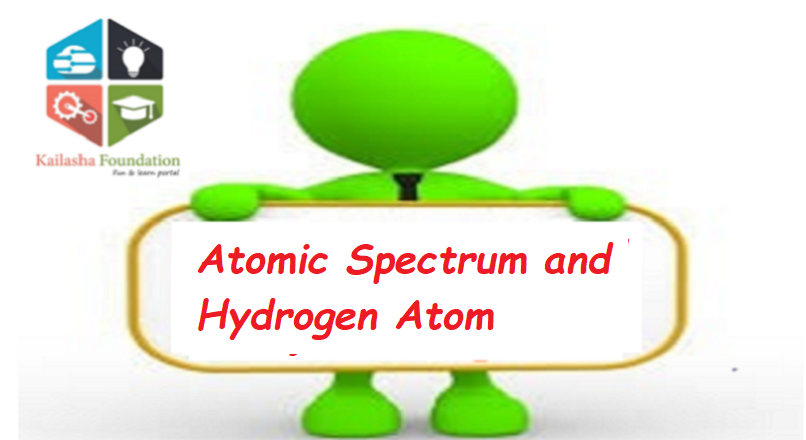## Register Now

Join now to get your problems solved with ease. Register with Email. Feel free to WhatsApp all issues on 8294600829.## Atomic Spectrum and Hydrogen Atom

### Wave nature of Electromagnetic Radiation

Properties of electromagnetic waves:

• Electromagnetic waves are composed of oscillating electric and magnetic field. The electric and magnetic field oscillate perpendicular to each other as well as to the direction of propagation of wave.
• Electromagnetic waves do not require a medium to travel.
• There are many electromagnetic waves which are characterized by their frequency (υ) or wavelength (λ). The whole set of electromagnetic waves is called electromagnetic spectrum.
• There is only a small part of electromagnetic spectrum, which is visible to our eyes and we call it visible region.
• The SI unit of frequency (υ) is hertz. Frequency is defined as the number of waves that pass through a given point in one second.
• The SI unit of wavelength (λ) is meter (m), however, smaller units of length are used for electromagnetic waves since they have very small wavelength.
• In vaccum, all electromagnetic waves travels with the same speed viz. speed of light i.e. 3.0 × 108 m/s. The speed of light (c), frequency (υ) and wavelength (λ) of an electromagnetic wave are related by c = υλ.

### Atomic Spectrum and Hydrogen Atom

If an electron in an atom gains energy, it reached to higher energy level from low energy level by absorbing energy. This indicated that a specific wavelength is absorbed by the atoms and a dark line is observed in the spectrum. This dark line constitutes absorption spectrum.

Similarly, if the electron jumps from higher energy level to lower energy level, amount of energy is released and corresponding to that energy, an electromagnetic wave of specific wavelength is emitted. This constitutes emission spectrum.

Hydrogen Spectrum:

If electric discharge is passed through hydrogen gas and the emitted radiation are analyzed then it is found to be consist of UV, Visible and IR regions. The series of lines is called as line or atomic spectrum of hydrogen.

Each line of the atomic spectrum corresponds to a light of definite wavelength. The entire spectrum consists of six series of lines each series, known after their discoverer as the Balmer, Paschen, Lyman, Brackett, Pfund and Humphrey series.

The wavelength of these series can be calculated by using the formulaWhere, λ = Wavelength, R = Rydberg’s Constant (109678 cm-1) and n1 and n2 have values as given below.

### Planck’s quantum theory:

A black body when heated, emits thermal radiation of different wavelengths (or frequency). To explain this, Max Planck gave this theory.

The main points were

• Atoms and molecules can emit or absorbs energy in discrete quantity and not in a continuous manner.
• The smallest of such discrete quantity of energy, that can be absorbed or emit, was called as quantum. In case of light, this quantum is called photon.
• The energy of quantum is proportional to the frequency of radiation.

So, E ∝ υ

Or, E = hυ where υ is the frequency of radiation and h is Planck’s constant. The value of h is 6.626 × 10-34 J-sec.

• A body can only radiate or emit energy in whole number multiple of this quantum i.e.  hν, 2hν, 3hν ….. nhν where n is the positive integer.